### IMO Shortlist 2010 problem A4

Kvaliteta:
Avg: 0,0
Težina:
Avg: 7,0
A sequence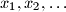$x_1, x_2, \ldots$ is defined by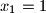$x_1 = 1$ and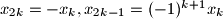$x_{2k}=-x_k, x_{2k-1} = (-1)^{k+1}x_k$ for all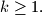$k \geq 1.$ Prove that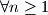$\forall n \geq 1$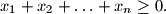$x_1 + x_2 + \ldots + x_n \geq 0.$

Proposed by Gerhard Wöginger, Austria
Izvor: Međunarodna matematička olimpijada, shortlist 2010